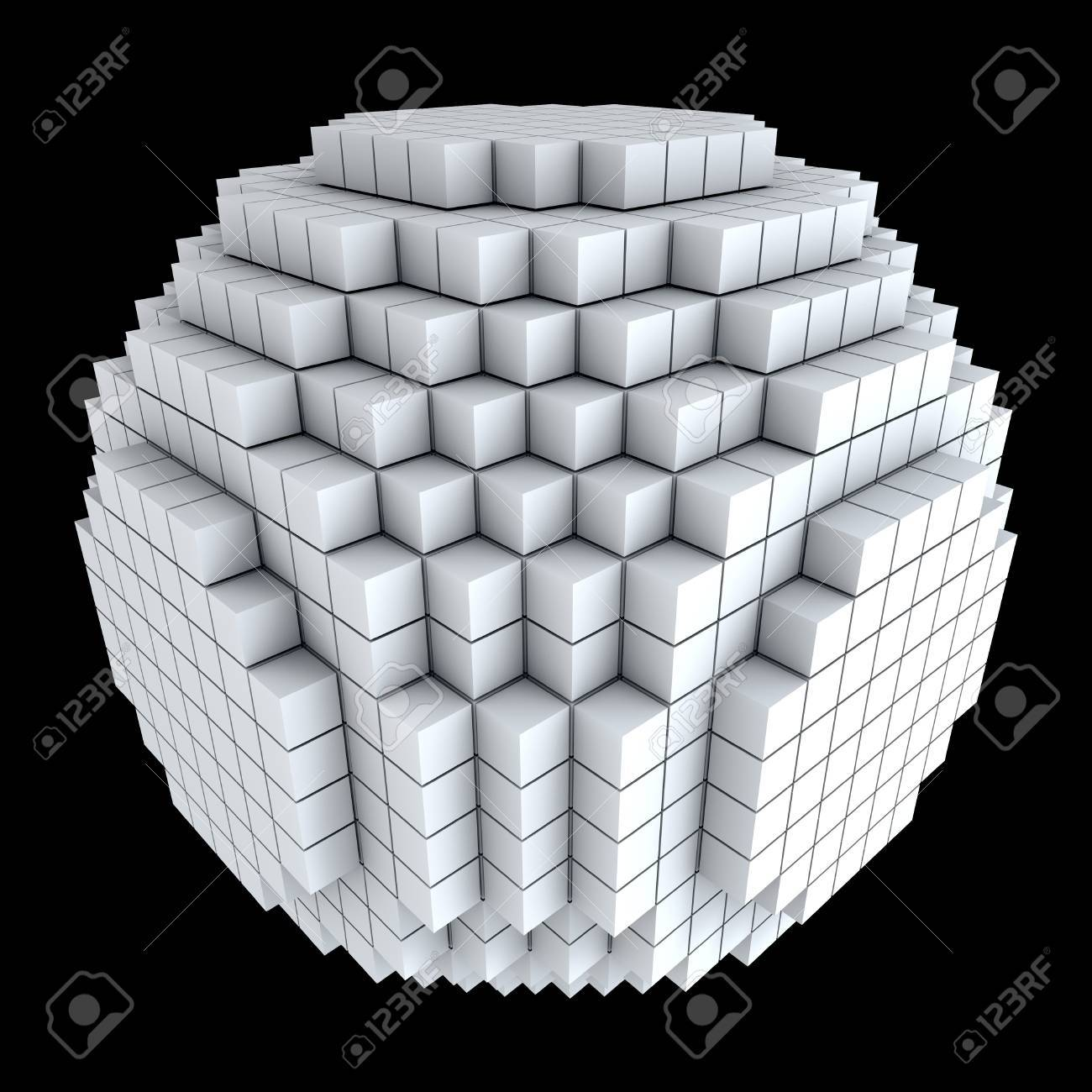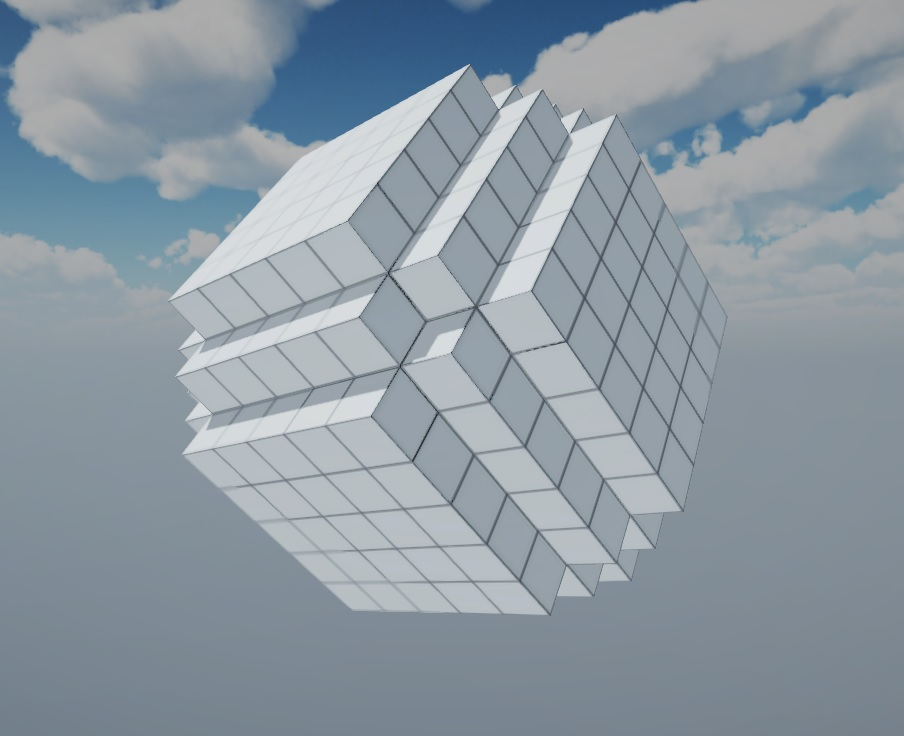# Code to create sphere out of cubes

Updated on January 7, 2020 in
2 on November 24, 2019

Hello everybody,

I am struggling at the moment to create a sphere out of cubes. My idea was the following:

• First, I create a loop which would create a 3D cube out of cubes.
• Second, I would skip the positions, which are not neccessary for the sphere.

It should look like this:So I just give as an input a diameter and the code generates a sphere out of blocks/cubes. But to code this is harder than I had imagined. I had tried a lot of different continue conditions, but nothing gets me the sphere as a result.

```private void CreateSphere(int diameter)
{
int radius = diameter / 2;
//create blocks in all three dimensions
for (int x = 0; x < diameter; x++)
{
for (int y = 0; y < diameter; y++)
{
for (int z = 0; z < diameter; z++)
{
//skip outer ring
if ((x < y || x >= diameter- y) || (z < y || z >= diameter- y))
continue;
CreateBlock(x, y, z);
}
}
}
}
```

So I am just asking, if you have a better idea how to do it?

• Liked by
on December 25, 2019

As you create cubes within your 3D region of space, first check that they are within the radius of the center of the sphere desired.

Note: it turns out that in order to check the distance between two points, square root calculations must be made, and that is an intensive calculation, so what’s more efficient, is to compare squares of distances, rather than pure distances.

```private void CreateSphere(int diameter,int sphereCenterX,int sphereCenterY,int sphereCenterZ)
{
int radius = diameter / 2;
Vector3 sphereCenter = new Vector3(sphereCenterX, sphereCenterY, sphereCenterZ);
//create blocks in all three dimensions
{
{
{
//new cube position
Vector3 newCubePos = new Vector3(x,y,z);
Vector3 vectorToNewCubeFromCenter = newCubePosition - sphereCenter;
CreateBlock(x, y, z);
}
}
}
}
}
```Helpful
on January 7, 2020

Thank you very much! After some tweeks it works pretty well.```private void CreateSphereMatrix()
{
int radius = matrixSize / 2;
Vector3 sphereCenter = Vector3.one * radius;
//create blocks in all three dimensions
for (int x = 0; x < matrixSize; x++)
{
for (int y = 0; y < matrixSize; y++)
{
for (int z = 0; z < matrixSize; z++)
{
//if new cube position is inside radisu square
Vector3 newCubePos = new Vector3(x, y, z);
Vector3 vectorToNewCubeFromCenter = newCubePos - sphereCenter;
{
CreateBlock(x, y, z);
}
}
}
}
}
```